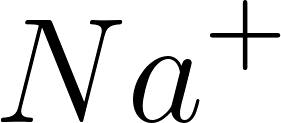×
Get Full Access to Introductory Chemistry - 5 Edition - Chapter 4 - Problem 81p
Get Full Access to Introductory Chemistry - 5 Edition - Chapter 4 - Problem 81p

×

Determine the number of protons and electrons in each ion.(a) Na+(b) Ba2+(c) O2?(d) Co3+ISBN: 9780321910295 34

Solution for problem 81P Chapter 4

Introductory Chemistry | 5th Edition

• Textbook Solutions
• 2901 Step-by-step solutions solved by professors and subject experts
• Get 24/7 help from StudySoup virtual teaching assistantsIntroductory Chemistry | 5th Edition

4 5 1 310 Reviews
29
1
Problem 81P

Problem 81P

Determine the number of protons and electrons in each ion.

(a) Na+

(b) Ba2+

(c) O2−

(d) Co3+

Step-by-Step Solution:

Problem 81P

Determine the number of protons and electrons in each ion.

(a) Na+

(b) Ba2+

(c) O2-

(d) Co3+

Step by Step Solution

Step 1 of 4

(a)

The given ion -Sodium atomic number - 11

Losing an electron from a neutral atom makes a positive charge ion.

Number of protons is equal to the atomic number of an element.

Number of protons inis 11.

Number of electrons inTherefore, the number of protons and electrons inis 11 and 10 respectively.

___________________________________________________________________________

Step 2 of 4

Step 3 of 4

ISBN: 9780321910295

Introductory Chemistry was written by and is associated to the ISBN: 9780321910295. This full solution covers the following key subjects: determine, electrons, ion, Protons. This expansive textbook survival guide covers 19 chapters, and 2046 solutions. Since the solution to 81P from 4 chapter was answered, more than 637 students have viewed the full step-by-step answer. The full step-by-step solution to problem: 81P from chapter: 4 was answered by , our top Chemistry solution expert on 05/06/17, 06:45PM. This textbook survival guide was created for the textbook: Introductory Chemistry, edition: 5. The answer to “Determine the number of protons and electrons in each ion.(a) Na+(b) Ba2+(c) O2?(d) Co3+” is broken down into a number of easy to follow steps, and 14 words.

Unlock Textbook Solution